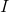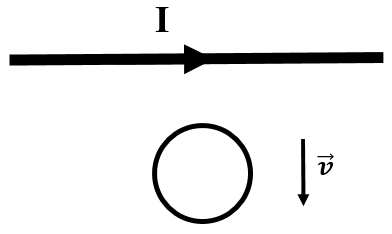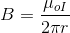# AP Physics C Electricity : Using Lenz's Law

## Example Questions

### Example Question #1 : Using Lenz's Law

A wire loop carrying no current is placed near another very long straight wire carrying current. The loop is then moved directly away from the straight wire at a constant speed.In which direction will the current induced in the small wire loop flow?

Counterclockwise

Clockwise

No current will be induced in the wire loop

Not enough information is given to solve

Clockwise

Explanation:

The magnetic field due to the long straight wire points into the page (right-hand rule) in the region below it in the picture. The magnitude of this magnetic field is given by:The magnetic field thus decreases in value with increasing distance,. As such, the amount of flux passing through the wire loop decreases steadily as it is moved away from the wire.

According to Lenz's Law, an induced current within the wire loop will counteract this loss of magnetic flux from the straight wire. The magnetic field from the induced current in the wire loop will consequently point in the same direction as that from the current in the straight wire. The right-hand rule for a magnetic field into the page yields an induced current direction in the clockwise direction.